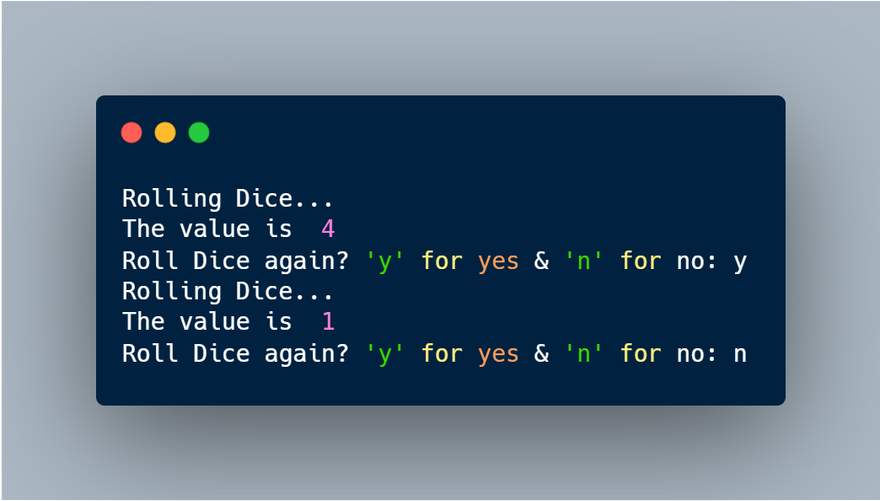## CodeNewbie Community 🌱# How to build a Dice Roller in Python

Hello everyone, today we are going to create a Dice Roller in Python.## How does it work?

In the real world, we use dice to get a random number ranging from 1- 6 so we can proceed with the game accordingly. Our program is going to work similarly. Every time we will run our program, it will return us a random number between 1 & 6. Now let's jump to coding and make this project.

## Let's Code

The first thing we are gonna do is import the required modules.

For this project, we need only one module which is `random` module which helps us to generate random values.

``````import random
``````

We have successfully imported `random` module.

Now we may need to use the Dice Roller multiple times hence it will be a good idea to use an `while` loop in order to keep our code running for as long as user wishes.

``````while True:
pass
``````

Here we go! Here is the basic syntax of the `while` loop. We will substitute that `pass` keyword with our remaining code.

Now let's give our code the ability to generate a random number between 1 to 6.

Here we will make use of one of the functions of our `random` module.

``````print(f"The value is ", random.randint(1,6))
``````

`random.randint(START_VALUE, END_VALUE)` is the function we are going to use.

As previously mentioned, this function will take an `START_VALUE` & an `END_VALUE` which will be `1` & `6` in our case, since we need a value between 1 & 6.

We are also using an `print()` function along with `f-string` to directly print the output on the console, rather than storing it in a variable.

We are almost done!

Now let's add one line to ask the user if he/she wishes to roll the dice again or would like the end the program instead. This is pretty simple.

``````repeat = input("Roll Dice again? 'y' for yes & 'n' for no: ")
if repeat == 'n':
break
``````

Here we are simply using input() function to ask the user and the user can reply in either yes or no. And we are using an `if` statement so that if the user replies no, then the loop will terminate otherwise the loop will continue to run forever.

Finally here's how our `while` loop will look like:

``````while True:
print("Rolling Dice...")
print(f"The value is ", random.randint(1,6))
repeat = input("Roll Dice again? 'y' for yes & 'n' for no: ")
if repeat == 'n':
break
``````

Here we have also added an optional `print()` function to indicate the rolling of the dice.

You did it! 🤩

## Source Code

You can find the complete source code of this project here -

mindninjaX/Python-Projects-for-Beginners

## Support

Thank you so much for reading! I hope you found this beginner project useful.

If you like my work please consider Buying me a Coffee so that I can bring more projects, more articles for you.